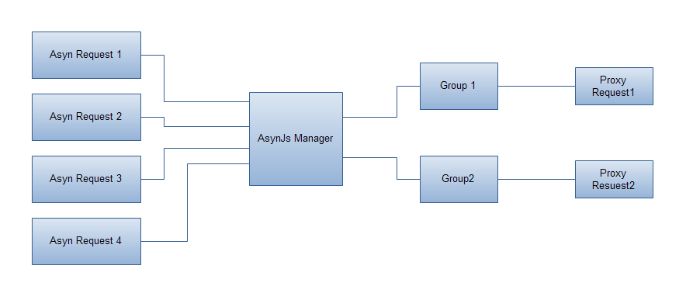09月07, 2010

# 如何减少前端异步请求的数量

## 实现方案## 实现代码

``````if (typeof Space == 'undefined') window.Space = {};
Space.asynJs = function() {
var _isStart = false,
stack = [];

function start() {
if (_isStart) {
throw new Error('asynJs request is start.');
return false;
}
_isStart = true;
for (var i = 0, len = stack.length; i < len; i++) {
if (!stack[i]) continue;
//如果该组只有一条记录，就不用走通用代理了。
if (stack[i].length == 1) {
baidu.sio.callByServer(decodeURIComponent(url));
} else {
var url = 'url[]=' + stack[i].join('&url[]=');
}
}
}

group = group | 0;
!stack[group] && (stack[group] = []);
stack[group].push(encodeURIComponent(url));
}
return {
start: start
}
}()``````

``````Space.asynJs.load('http://pageurl1', 1)
//在页面最底部启动请求
Space.asynJs.start();``````

## PHP端的实现

``````<?php
//url is array
\$urls = \$_GET['url'];
//经过白名单过滤后的URL
\$urls = apply_domain_filter(\$urls);
//实例化Curl类
\$curl = new Curl();
\$contents = array();
//这里实现的时候可以使用并发处理
foreach(\$urls as \$url){
//需要配置对应URL的transmit
}
echo join(';', \$contents);
?>``````